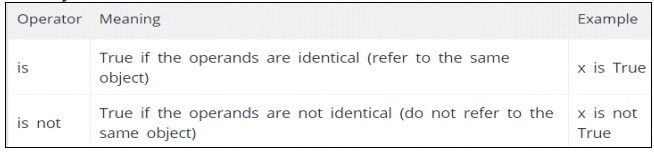Home | | Problem Solving and Python Programming | Operators and Types of Operators - Python

# Operators and Types of Operators - Python

Operators are the constructs which can manipulate the value of operands.

## OPERATORS:

v   Operators are the constructs which can manipulate the value of operands.

v   Consider the expression 4 + 5 = 9. Here, 4 and 5 are called operands and + is called operator

## Types of Operators:

-Python language supports the following types of operators

·                 Arithmetic Operators

·                 Comparison (Relational) Operators

·                 Assignment Operators

·                 Logical Operators

·                 Bitwise Operators

·                 Membership Operators

·                 Identity Operators

## Arithmetic operators:

They are used to perform mathematical operations like addition, subtraction, multiplication etc. Assume, a=10 and b=5### Examples

a=10

b=5

print("a+b=",a+b)

print("a-b=",a-b)

print("a*b=",a*b)

print("a/b=",a/b)

print("a%b=",a%b)

print("a//b=",a//b)

print("a**b=",a**b)

a+b= 15

a-b= 5

a*b= 50

a/b= 2.0

a%b= 0

a//b= 2

a**b= 100000

## Comparison (Relational) Operators:

·                 Comparison operators are used to compare values.

·                 It either returns True or False according to the condition. Assume, a=10 and b=5### Example

a=10

b=5

print("a>b=>",a>b)

print("a>b=>",a<b)

print("a==b=>",a==b)

print("a!=b=>",a!=b)

print("a>=b=>",a<=b)

print("a>=b=>",a>=b)

a>b=> True

a>b=> False

a==b=> False

a!=b=> True

a>=b=> False

a>=b=> True

## Assignment Operators:

-Assignment operators are used in Python to assign values to variables.### Example

a = 21

b = 10

c = 0

c = a + b

print("Line 1 - Value of c is ", c)

c += a

print("Line 2 - Value of c is ", c)

c *= a

print("Line 3 - Value of c is ", c)

c /= a

print("Line 4 - Value of c is ", c)

c= 2 c %= a

print("Line 5 - Value of c is ", c) c **= a

print("Line 6 - Value of c is ", c) c //= a

print("Line 7 - Value of c is ", c)

### Output

Line 1 - Value of c is 31

Line 2 - Value of c is 52

Line 3 - Value of c is 1092

Line 4 - Value of c is 52.0

Line 5 - Value of c is 2

Line 6 - Value of c is 2097152

Line 7 - Value of c is 99864

## Logical Operators:

-Logical operators are the and, or, not operators.Example

a = True

b = False

print('a and b is',a and b)

print('a or b is',a or b)

print('not a is',not a)

x and y is False

x or y is True

not x is False

## Bitwise Operators:

A bitwise operation operates on one or more bit patterns at the level of individual Bits

### Example:

Let x = 10 (0000 1010 in binary) and

y = 4 (0000 0100 in binary)Example

a = 60      # 60 = 0011 1100

b = 13     # 13 = 0000 1101

c = 0

c = a & b;      # 12 = 0000 1100

print "Line 1 - Value of c is ", c

c = a | b;  # 61 = 0011 1101

print "Line 2 - Value of c is ", c

c = a ^ b; # 49 = 0011 0001

print "Line 3 - Value of c is ", c

c = ~a;    # -61 = 1100 0011

print "Line 4 - Value of c is ", c

c = a << 2;     # 240 = 1111 0000

print "Line 5 - Value of c is ", c

c = a >> 2;     # 15 = 0000 1111

print "Line 6 - Value of c is ", c

### Output

Line 1 - Value of c is 12

Line 2 - Value of c is 61

Line 3      - Value of c is 49

Line 4      - Value of c is -61

Line 5      - Value of c is 240

Line 6      - Value of c is 15

## Membership Operators:

v   Evaluates to find a value or a variable is in the specified sequence of string, list, tuple, dictionary or not.

v   Let, x=[5,3,6,4,1]. To check particular item in list or not, in and not in operators are used.x=[5,3,6,4,1]

>>>  5 in x

True

>>>  5 not in x

False

## Identity Operators

They are used to check if two values (or variables) are located on the same part of the memory.### Example

x = 5

y = 5

x2 = 'Hello'

y2 = 'Hello'

print(x1 is not y1)

print(x2 is y2)

### Output

False

True

Study Material, Lecturing Notes, Assignment, Reference, Wiki description explanation, brief detail
Problem Solving and Python Programming : Data, Expressions, Statements : Operators and Types of Operators - Python |